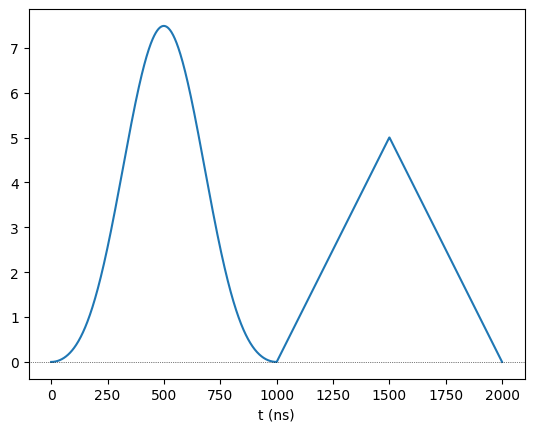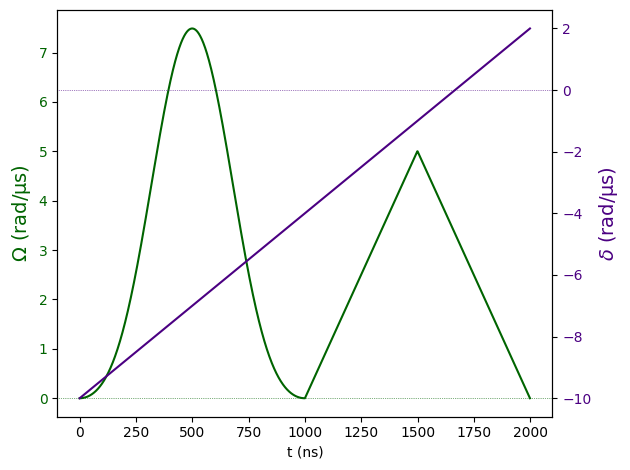# Composite Waveforms

:

import numpy as np

from pulser import Pulse
from pulser.waveforms import (
BlackmanWaveform,
RampWaveform,
CompositeWaveform,
ConstantWaveform,
)


When creating a Sequence, it’s usually preferable to divide it into a stream of simple pulses. However, this is not always convenient, as the natural breaking point in the amplitude and detuning waveforms may not always match.

In these cases, the CompositeWaveform allows for the creation of a more complex waveform by concatenation of multiple, smaller waveforms. Here’s an example of how to use it:

:

# Defining simple waveforms
pi_pulse = BlackmanWaveform(1000, np.pi)  # Blackman pi-pulse of 1us
up = RampWaveform(500, 0, 5)
down = RampWaveform(500, 5, 0)

# Creating the CompositeWaveform
comp_wf = CompositeWaveform(pi_pulse, up, down)
comp_wf.draw()

# All usual metrics are still availabe
print("Composite pulse duration: ", comp_wf.duration)
print("Composite pulse area: ", comp_wf.integral)Composite pulse duration:  2000
Composite pulse area:  5.641592653589793


Say that this waveform modulates the amplitude, but alongside it we would like a detuning in the form of a RampWaveform, going from $$-10$$ to $$2$$ rad/µs. Without the composite waveform, we would be forced to calculate the detuning values at $$t=1000$$ ns and $$t=1500$$ ns, in order to create three separate ramps. Instead, we can make a single ramp and use it to create a single pulse:

:

detuning_ramp = RampWaveform(comp_wf.duration, -10, 2)

pulse = Pulse(comp_wf, detuning_ramp, phase=0)
pulse.draw()